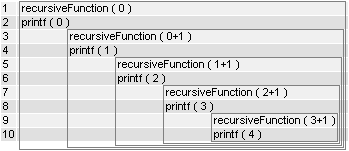# Recursive function

recursive functions in c# .net with example of fibonacci sequence

In simplest possible way, recursive function is a function that calls itself. It’s common for function to call another function, but when it calls itself its a recursive function.  Recursive method calls itself so many times until being satisfied, this method should call itself with new parameters to avoid infinite loop.We will use Fibonacci sequence as an an example.

There are two ways of creating Fibonacci  sequence in as an recursive function. We will take a look at both of them.

This is the first option with three parameters, first two represent numbers to add, third is a counter of iterations, last one is passed by the user and represent the length of the series.

```private static void FibonacciSequenceTemp(int a, int b, int counter,int len)
{
if (counter <= len)
{
Console.Write(a + "  ");
FibonacciSequenceTemp(b, a + b, counter+1, len);
}
}```

We can call this function directly or to simplify the interface we can create a function with one input parameter.

```public static void FibonacciSequence(int len)
{
FibonacciSequenceTemp(0, 1, 1, len);
}```

This way the function can be executed with different parameters each iteration, and we don’t need to specify conditions for low iteration numbers like 0 or 1, which we will do in another example;

Another way of dealing with this problem is to create with function with one input that will be called twice and will represent numbers to add. In this example we need to include conditions like n=0 or n=1, because this function works only for argument grater than one

```

static void Main(string[] args)
{
int n, i = 0;
//n is a number of elements
n=5;
//loop for pinting all values
for ( int c = 1 ; c <= n ; c++ )
{
Console.WriteLine(Fibonacci(i));
i++;
}
Console.ReadKey();
}
static int Fibonacci(int n)
{
if (n == 0)
return 0;
else if (n == 1)
return 1;
else
return (Fibonacci(n - 1) + Fibonacci(n - 2));
}
```

In both examples we are calling the function within itself, what makes it recursive.  we need to remember to change the input parameters with every iteration to not stuck in infinite loop. Fibonacci sequence can be created without using recursive functions, but it is a good demonstration of practical use of it. In next article I’ll cover  another ways of dealing with Fibonacci sequence. Stay Awesome!## Author: indiedevart

Indie Games Developer